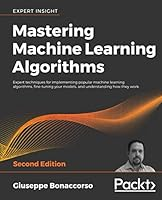# Mastering Machine Learning Algorithms, 2nd Edition## Book Description

Updated and revised second edition of the bestselling guide to exploring and mastering the most important algorithms for solving complex problems

#### Key Features

• Updated to include new algorithms and techniques
• Code updated to Python 3.8 & TensorFlow 2.x
• New coverage of regression analysis, time series analysis, deep learning models, and cutting-edge applications

#### Book Description

Mastering Machine Learning Algorithms, Second Edition helps you harness the real power of machine learning algorithms in order to implement smarter ways of meeting today's overwhelming data needs. This newly updated and revised guide will help you master algorithms used widely in semi-supervised learning, reinforcement learning, supervised learning, and unsupervised learning domains.

You will use all the modern libraries from the Python ecosystem – including NumPy and Keras – to extract features from varied complexities of data. Ranging from Bayesian models to the Markov chain Monte Carlo algorithm to Hidden Markov models, this machine learning book teaches you how to extract features from your dataset, perform complex dimensionality reduction, and train supervised and semi-supervised models by making use of Python-based libraries such as scikit-learn. You will also discover practical applications for complex techniques such as maximum likelihood estimation, Hebbian learning, and ensemble learning, and how to use TensorFlow 2.x to train effective deep neural .

By the end of this book, you will be ready to implement and solve end-to-end machine learning problems and use case scenarios.

#### What you will learn

• Understand the characteristics of a machine learning algorithm
• Implement algorithms from supervised, semi-supervised, unsupervised, and RL domains
• Learn how regression works in time-series analysis and prediction
• Create, model, and train complex probabilistic models
• Cluster high-dimensional data and evaluate model accuracy
• Discover how artificial neural networks work – train, optimize, and validate them
• Work with autoencoders, Hebbian networks, and GANs

#### Who this book is for

This book is for data science professionals who want to delve into complex ML algorithms to understand how various machine learning models can be built. Knowledge of Python programming is required.

1. Machine Learning Model
2. Loss functions and Regularization
3. Introduction to Semi-Supervised Learning
5. -based Semi-Supervised Learning
6. Clustering and Unsupervised Models
7. Advanced Clustering and Unsupervised Models
8. Clustering and Unsupervised Models for
9. Generalized Linear Models and Regression
10. Introduction to Time-Series Analysis
11. Bayesian Networks and Hidden Markov Models
12. The EM Algorithm
13. Component Analysis and Dimensionality Reduction
14. Hebbian Learning
15. Fundamentals of Ensemble Learning
17. Modeling Neural Networks
18. Optimizing Neural Networks
19. Deep Convolutional Networks
20. Recurrent Neural Networks
21. Auto-Encoders
22. Introduction to Generative Adversarial Networks
23. Deep Belief Networks
24. Introduction to Reinforcement Learning

## Book Details

• Title: Mastering Machine Learning Algorithms, 2nd Edition
• Author:
• Length: 798 pages
• Edition: 1
• Language: English
• Publisher:
• Publication Date: 2020-01-31
• ISBN-10: 1838820299
• ISBN-13: 9781838820299